# Confidence Intervals with proportions a k a 1

• Slides: 23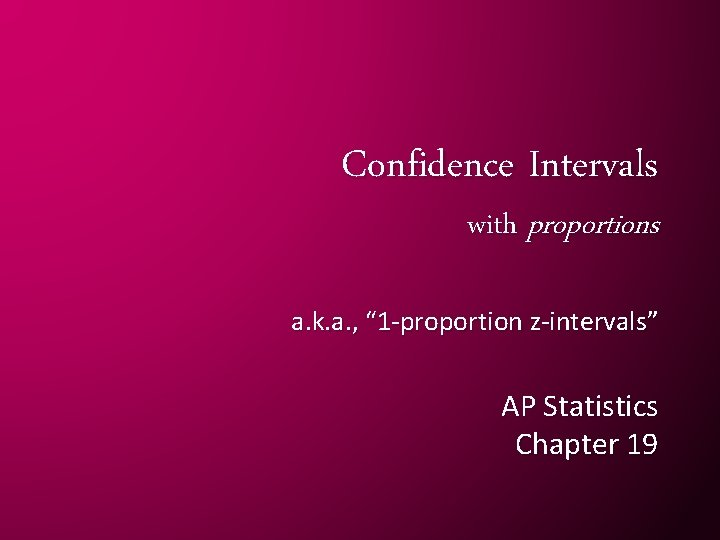Confidence Intervals with proportions a. k. a. , “ 1 -proportion z-intervals” AP Statistics Chapter 191 -proportion z-interval Statistic + Critical value × Standard deviation of the statistic POINT ESTIMATE STANDARD ERROR (an estimate for std. dev)CONDITIONS: Check these EVERY TIME you construct a CI!!! • Random sample? • 10% rule? • Success/failure: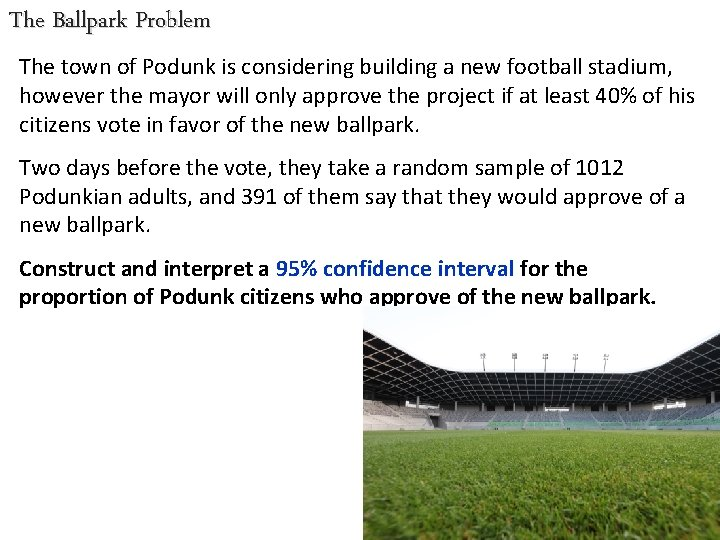The Ballpark Problem The town of Podunk is considering building a new football stadium, however the mayor will only approve the project if at least 40% of his citizens vote in favor of the new ballpark. Two days before the vote, they take a random sample of 1012 Podunkian adults, and 391 of them say that they would approve of a new ballpark. Construct and interpret a 95% confidence interval for the proportion of Podunk citizens who approve of the new ballpark.The Ballpark Problem Two days before the vote, they take a random sample of 1012 Podunkian adults, and 391 of them say that they would approve of a new ballpark. Construct and interpret a 95% confidence interval for the proportion of Podunk citizens who approve of the new ballpark. p = true proportion of all Podunk adults that favor the stadium 1 -proportion z-interval Conditions: • Given that we have a random sample of Podunk citizens • 1012 is (hopefully) less than 10% of all adults in Podunk* • Success/failure: We are 95% confident that the true proportion of Podunkians that are in favor of the new stadium is between 35. 64% and 41. 64%. *or else would they really need a new stadium? ? ?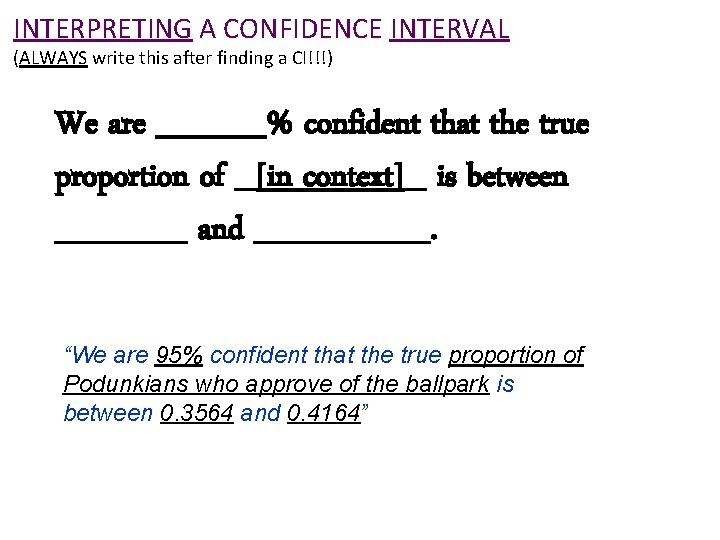INTERPRETING A CONFIDENCE INTERVAL (ALWAYS write this after finding a CI!!!) We are _____% confident that the true proportion of _[in context]_ is between ______ and ____. “We are 95% confident that the true proportion of Podunkians who approve of the ballpark is between 0. 3564 and 0. 4164”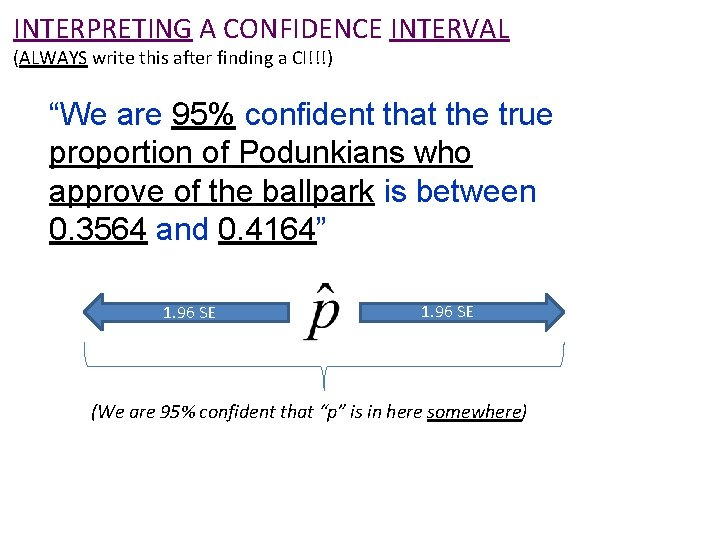INTERPRETING A CONFIDENCE INTERVAL (ALWAYS write this after finding a CI!!!) “We are 95% confident that the true proportion of Podunkians who approve of the ballpark is between 0. 3564 and 0. 4164” 1. 96 SE (We are 95% confident that “p” is in here somewhere)INTERPRETING CONFIDENCE LEVEL (only do this when asked) • If we collect a LARGE number of samples using this method, about ____% of the resulting confidence intervals will contain the true [proportion/mean in context] (be sure to write in CONTEXT!!!) If we take a large number of samples using this method, about 95% of the resulting confidence intervals will contain the true proportion of Podunkians who approve of the new ballpark.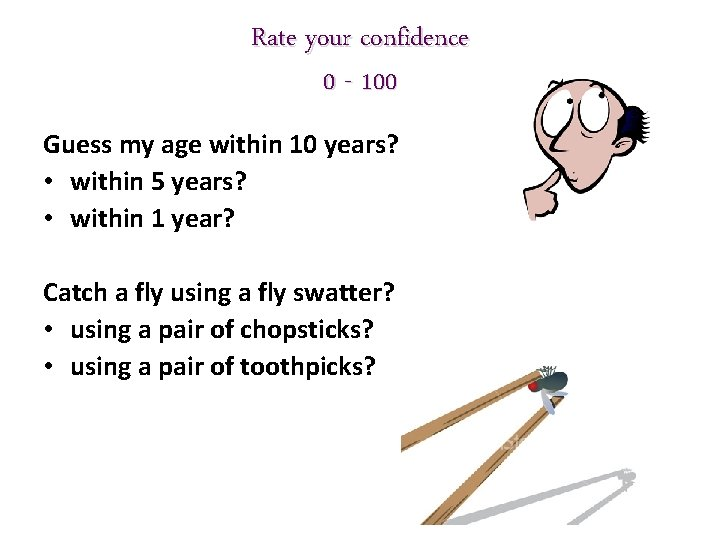Rate your confidence 0 - 100 Guess my age within 10 years? • within 5 years? • within 1 year? Catch a fly using a fly swatter? • using a pair of chopsticks? • using a pair of toothpicks?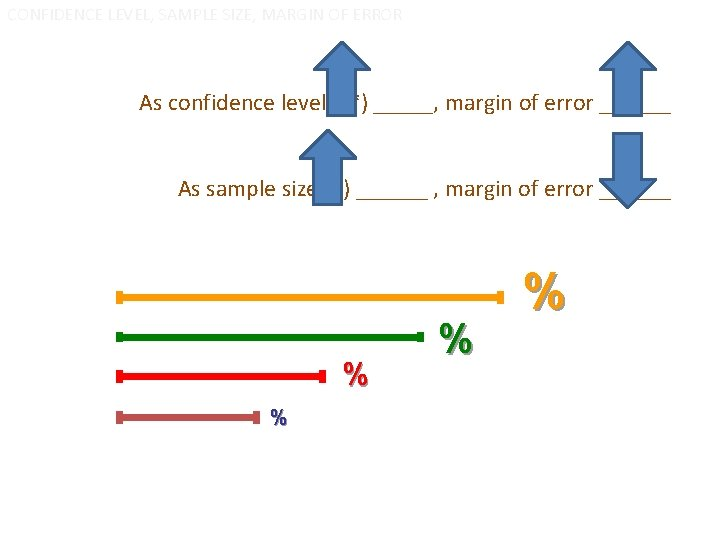CONFIDENCE LEVEL, SAMPLE SIZE, MARGIN OF ERROR As confidence level (z*) _____, margin of error ______ As sample size (n) ______ , margin of error ______ % %Critical value (z*) • Divide the tail area in HALF • Find the z-score for that percentile (take absolute value) Confidence level tail area z* 90%. 05 1. 645 95%. 025 1. 96 99%. 005 2. 576 For instance, for 90% confidence, you cut off 5% (or 0. 05) in EACH tail. The z-score to give you this is 1. 645. z*=1. 645. 05[fin]Confidence Intervals with proportions a. k. a. , “ 1 -proportion z-intervals” (part ii)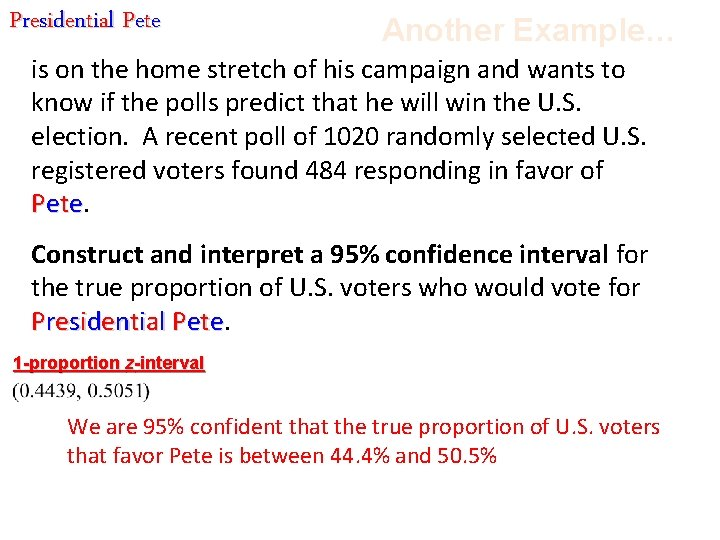Presidential Pete Another Example… is on the home stretch of his campaign and wants to know if the polls predict that he will win the U. S. election. A recent poll of 1020 randomly selected U. S. registered voters found 484 responding in favor of Pet e. Construct and interpret a 95% confidence interval for the true proportion of U. S. voters who would vote for P r e s i d e n t i al P e t e. 1 -proportion z-interval We are 95% confident that the true proportion of U. S. voters that favor Pete is between 44. 4% and 50. 5%confidence INTERVAL vs. confidence LEVEL 95% Confidence Interval: Interpreting the Confidence Interval: s!!! i h t o d S Y A W AL We are 95% confident that the true proportion of U. S. voters that favor Pete is between 44. 4% and 50. 5% Interpreting the Confidence Level: d. aske n e h w s i h t o d Using this method, about 95% of all samples would produce an interval that captures the true proportion of all U. S. voters that are in favor of Pete.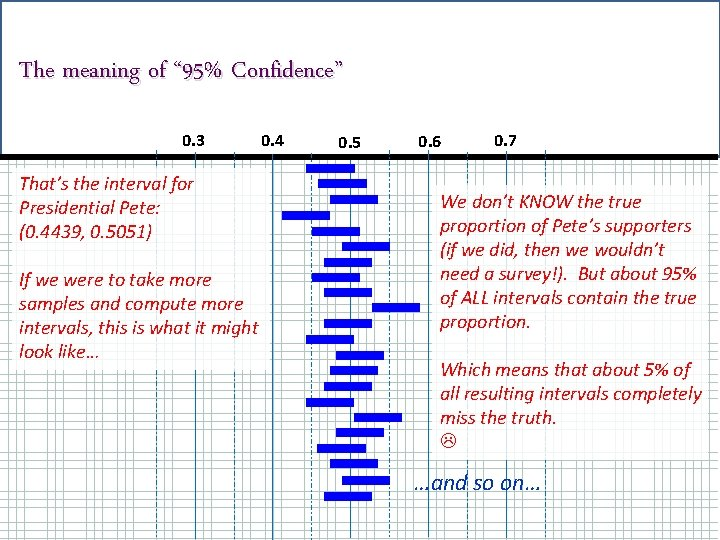The meaning of “ 95% Confidence” 0. 3 That’s the interval for Presidential Pete: (0. 4439, 0. 5051) If we were to take more samples and compute more intervals, this is what it might look like… 0. 4 0. 5 0. 6 0. 7 We don’t KNOW the true proportion of Pete’s supporters (if we did, then we wouldn’t need a survey!). But about 95% of ALL intervals contain the true proportion. Which means that about 5% of all resulting intervals completely miss the truth. …and so on…Hmmm… (questions to ponder) 1. What does the interval mean? Is Presidential Pete going to win? ? We don’t know, because the interval contains values both above and below 50% 2. How would raising the confidence level to 98% change the interval? This makes the interval wider (the bigger the margin of error, the more confidence you have – think the bigger the fly swatter…) 3. Pete wants another poll taken but only has enough campaign money left to poll 500 voters. What would this change in sample size do to the interval? This also makes the interval wider – “n” is in the denominator of the formula – dividing by a smaller number makes the margin of error bigger/wider.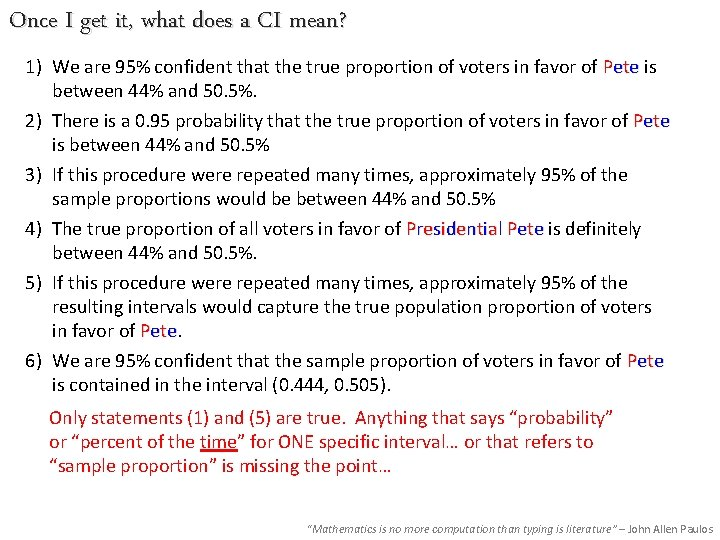Once I get it, what does a CI mean? 1) We are 95% confident that the true proportion of voters in favor of Pete is between 44% and 50. 5%. 2) There is a 0. 95 probability that the true proportion of voters in favor of Pete is between 44% and 50. 5% 3) If this procedure were repeated many times, approximately 95% of the sample proportions would be between 44% and 50. 5% 4) The true proportion of all voters in favor of Presidential Pete is definitely between 44% and 50. 5%. 5) If this procedure were repeated many times, approximately 95% of the resulting intervals would capture the true population proportion of voters in favor of Pete. 6) We are 95% confident that the sample proportion of voters in favor of Pete is contained in the interval (0. 444, 0. 505). Only statements (1) and (5) are true. Anything that says “probability” or “percent of the time” for ONE specific interval… or that refers to “sample proportion” is missing the point… “Mathematics is no more computation than typing is literature” – John Allen Paulos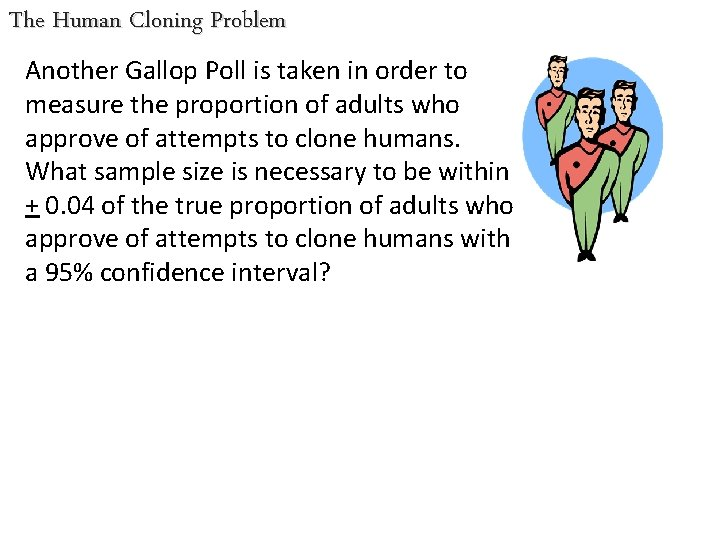The Human Cloning Problem Another Gallop Poll is taken in order to measure the proportion of adults who approve of attempts to clone humans. What sample size is necessary to be within + 0. 04 of the true proportion of adults who approve of attempts to clone humans with a 95% confidence interval?What p-hat (p) do you use when trying to find the sample size for a given margin of error? . 1(. 9) =. 09. 2(. 8) =. 16. 3(. 7) =. 21. 4(. 6) =. 24. 5(. 5) =. 25 By using 0. 5 for p-hat, we are using the worst-case scenario and using the largest SD in our calculations.What sample size is necessary to be within +0. 04 of the true proportion of adults who approve of attempts to clone humans with a 95% confidence interval? Use p-hat =. 5 Divide by 1. 96 Square both sides Round up on sample sizeHigh schoolers with cell phones According to an article in the Chicago Tribune, about 75% of all high school students owned a cell phone in 2010. It is reasonable to believe that since that time, this proportion has increased. Suppose we wish to conduct a survey to estimate the current proportion of high school students that have a cell phone within 6% with 90% confidence. Find the necessary sample size. (in this problem, we have additional information about the proportion of interest… so we should not use 0. 5 for p-hat)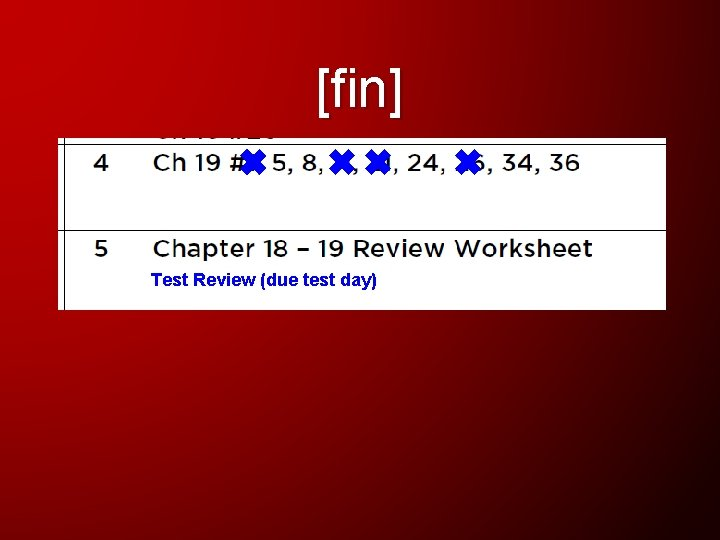[fin] Test Review (due test day)